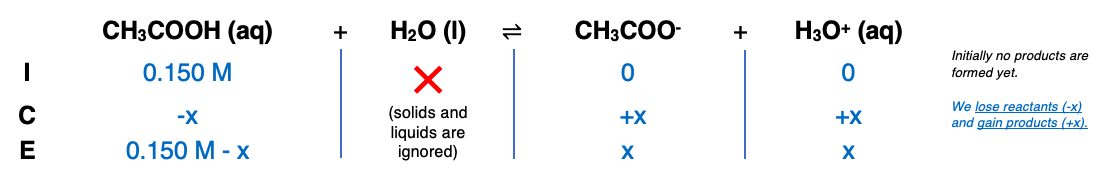# Problem: A 35.0-mL sample of 0.150 M acetic acid (CH3COOH) is titrated with 0.150 M  NaOH solution. Calculate the pH after the following volumes of base have been added.0 mL of the base

🤓 Based on our data, we think this question is relevant for Professor Goldberg's class at UPENN.

###### FREE Expert Solution###### Problem Details

A 35.0-mL sample of 0.150 M acetic acid (CH3COOH) is titrated with 0.150 M  NaOH solution. Calculate the pH after the following volumes of base have been added.

0 mL of the base